}

# How deMorgan's Theorems Can Help Programmers

Logic is an important aspect of programming and two important theorems in logic can be a big help to programmers.

#### Basics of computer logic

In software, logic is generally found in an if statement. This implements the idea that if some condition is true, then do something. For example,

If the user clicks a link then display that web page

`if a equals 10 then b = 7 or more often if (a==10) b=7`

And these can be more complex by using the ideas of and and or. For "x and y" both x and y must be true; likewise for "x or y" either can be true. It is just like the words in a natural language such as English.

These can be expressed visually with truth tables or Venn diagrams: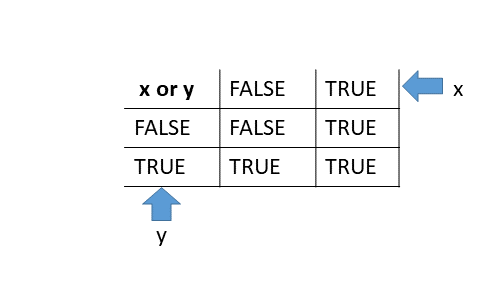Here, the top row and left column show the inputs: the values of x and y in our example. Both have to be true for the value of and to be true. It's like the addition tables use to teach children to add.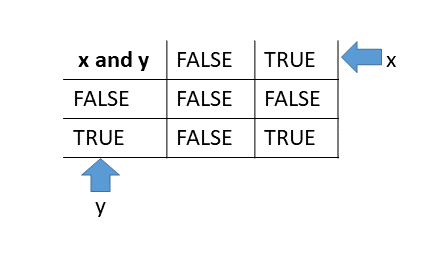Here, the top row and left column show the inputs: the values of x and y in our example. Only one has to be true for the value of or to be true.

With Venn diagrams: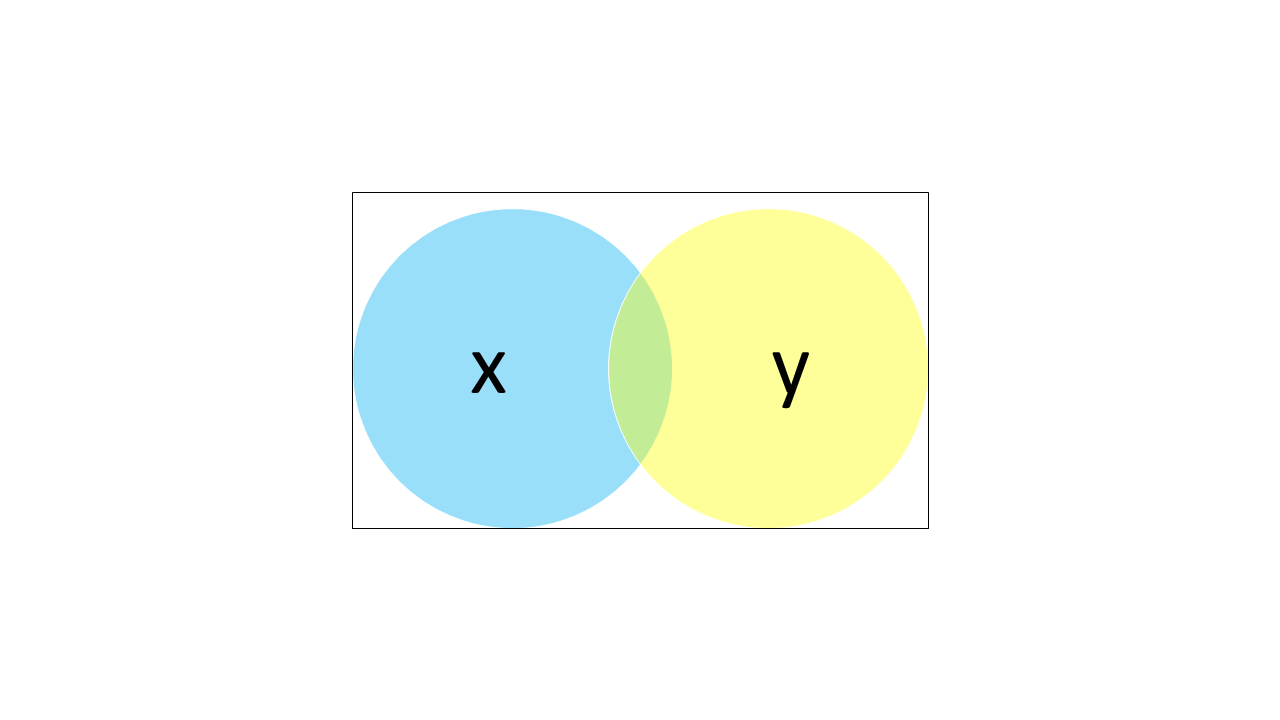Here all the colored are is the or and the green area where the circles overlap is the and. There is an additional operation called not. If we talk about "not x" we mean everything that is not in the blue circle. That includes all the white space and all the yellow that does not overlap with the blue "x" circle.

#### Complex expressions

The operators and, not and or are fairly straightforward. The issues come when we combine them.

Consider the case where "x is even and x is greater than 0" (so x is 2, 4, 6, 8, and so on). What if I wanted to exclude those positive even numbers? I could say "not 'x is even and x is greater than 0'", but many programmers would consider that ugly and difficult to read.

`even(x) and x > 0not (even(x) and x>0)`

[the parens are for grouping here]

`What we mean by "not 'x is even and x is greater than 0'" is really "x is odd or x is less than or equal to zero". What we did is apply one of deMorgan's theorems: we changed the senses of the comparisons ('even' became 'odd', and 'greater than zero' became less than or equal to zero') and we changed and to or. Our pseudocode then becomes odd(x) or x<= 0. That is generally believed to be more clear.`

Here are both of deMorgan's theorems: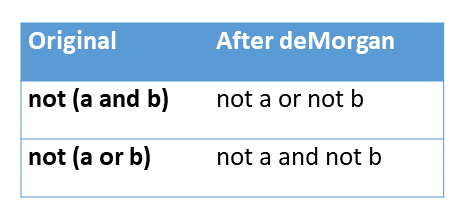And some examples: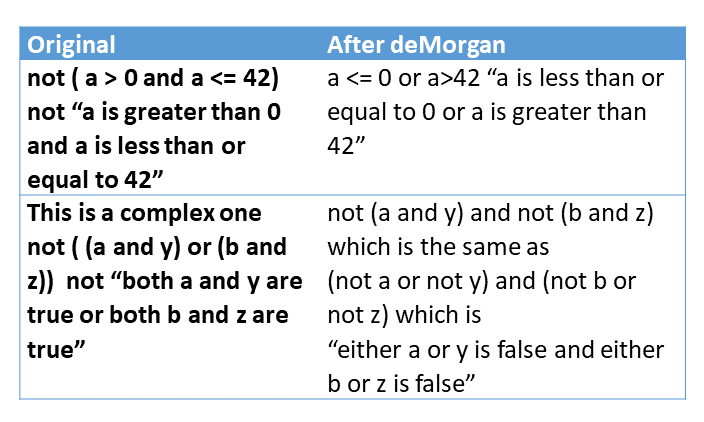These theorems are simple yet powerful. I hope you can use them to make your code more readable!

Chat With Us